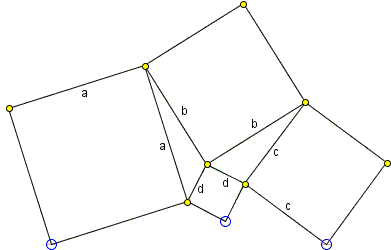# Four Hinged Squares

The purpose of the applet below is to illustrate an 1826 sangaku hung by Ikeda Sadakazu in an Azabu shrine, Tokyo. The tablet since disappeared but not before it was recorded in an 1827 book Shamei Sanpu (Sacred Mathematics) by Shiraishi Nagatada (1795-1862). The problem has also been included in an 1840 collection Sanpo Chokujutsu Seikai (Mathematics without Proof) by Heinouchi Masaomi.

Four squares are hinged as shown. When points A, B, C are collinear, what is the relationship between the sides of squares BEKH and KINS?

I first stumbled upon this simple problem in the Temple Geometry by Fukagawa and Pedoe, where it was listed without solution. My solution that employs Bottema's theorem appears on a separate page. Michel Cabart gave a solution that employed complex numbers. The newer book Sacred Geometry by Fukagawa and Rothman also lists the problem but now with the original solution from Sanpo Chokujutsu Seikai where it read:

Draw the three dashed squares; and contemplate the figure in detail; the result is trivial.

Fukagawa and Rothman give a solution nonetheless.

Shamei Sanpu in general contains by far more difficult problems. For example, the page where the four hinged squares is presented also includes a problem of determining the surface area of an ellipsoid:### References

1. H. Fukagawa, D. Pedoe, Japanese Temple Geometry Problems, The Charles Babbage Research Center, Winnipeg, 1989

Write to:

Charles Babbage Research Center
P.O. Box 272, St. Norbert Postal Station
Winnipeg, MB

2. H. Fukagawa, A. Rothman, Sacred Geometry: Japanese Temple Geometry, Princeton University Press, 2008, p. 149Square BEKH is inscribed in square UVTR so that

HV = KT

(and also EU = KR.) In the square circumscribing square CYIH,

HV = IQ

implying KT = IQ. Let M be the intersection of QT and IK. Then triangles KTM and IQM are congruent so that M is the midpoint of IK (KM = IM). Similarly, on the other side, KL = SL.

We conclude that since KM = KL and angle LKM is right while angle RKT is straight, triangles LRK and KTM are congruent. In square UVTR, KR = HT. So it follows that triangles LRK and KTH are also congruent leading to

Δ KTH = Δ KTM

and KM = KH. Just what was needed.

Incidently, there is another sangaku with a similar configuration:This has been written in 1832 also in the Tokyo prefecture and was recorded in Kokon Sankan by Uchida Gokan [Temple Geometry, p. 131]. The problem is to express d in terms of a, b, c. The solution is more conventional. Apply the Law of Cosines to triangles abd and bcd:

a² = b² + d² - 2bd·X
c² = b² + d² + 2bd·X,

where X is the cosine of the angle between b and d in triangle abd.

2d² = a² + c² - 2b²,

from which d is found to be

d = ½(a² + c² - 2b²).## Sangaku